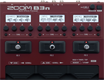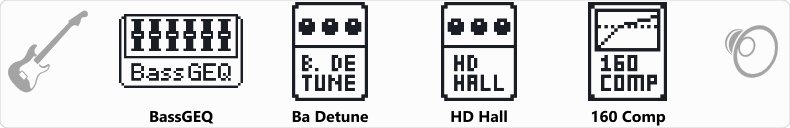# Factory : Harmonics

Discussion in 'Zoom B3n' started by Tonelib, May 20, 2018.

1. Factory : HarmonicsDevice: Zoom B3n
Firmware: 2.00

Name on device: Harmonics
Optimized for: Phones/Speaker

Effects chain:A subtle pitch shift and compression will enhance you playing of harmonics.

Effect: "BassGEQ" (Filter), active - "yes"
"50Hz" = 0.0
"120Hz" = 0.0
"400Hz" = 0.0
"500Hz" = 0.0
"800Hz" = 0.0
"4.5kHz" = 0.0
"10kHz" = 0.0
"Volume" = 80
"50Hz" = 0.0
"120Hz" = 0.0
"400Hz" = 0.0
"500Hz" = 0.0
"800Hz" = 0.0
"4.5kHz" = 0.0
"10kHz" = 0.0
"Volume" = 80
"Parameter set" = A

Effect: "Ba Detune" (Modulation), active - "yes"
"Cent" = 14
"PreD" = 1
"Tone" = 3
"Mix" = 85

Effect: "HD Hall" (Reverb), active - "yes"
"PreD" = 81
"Decay" = 45
"Mix" = 62
"Tail" = Off

Effect: "160 Comp" (Dynamics), active - "yes"
"Threshold" = -6
"Ratio" = 4.6
"Knee" = HARD
"Volume" = 46

Patch Volume: 100